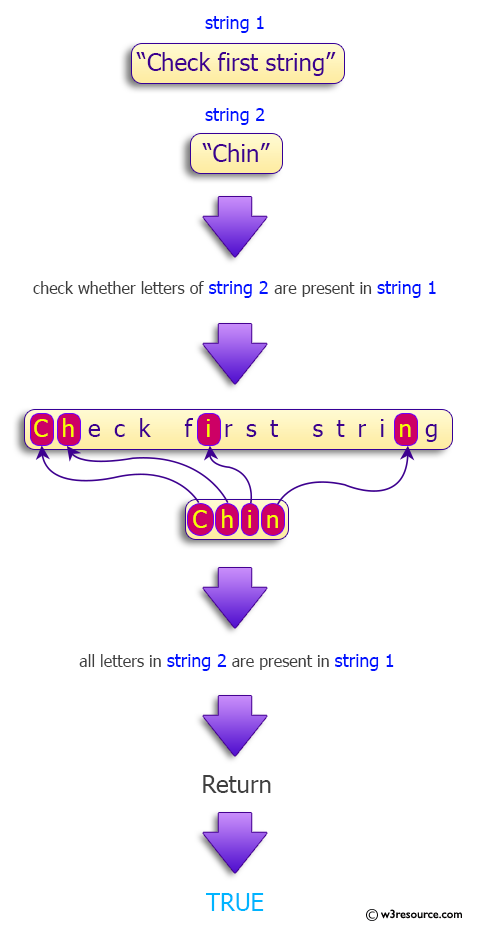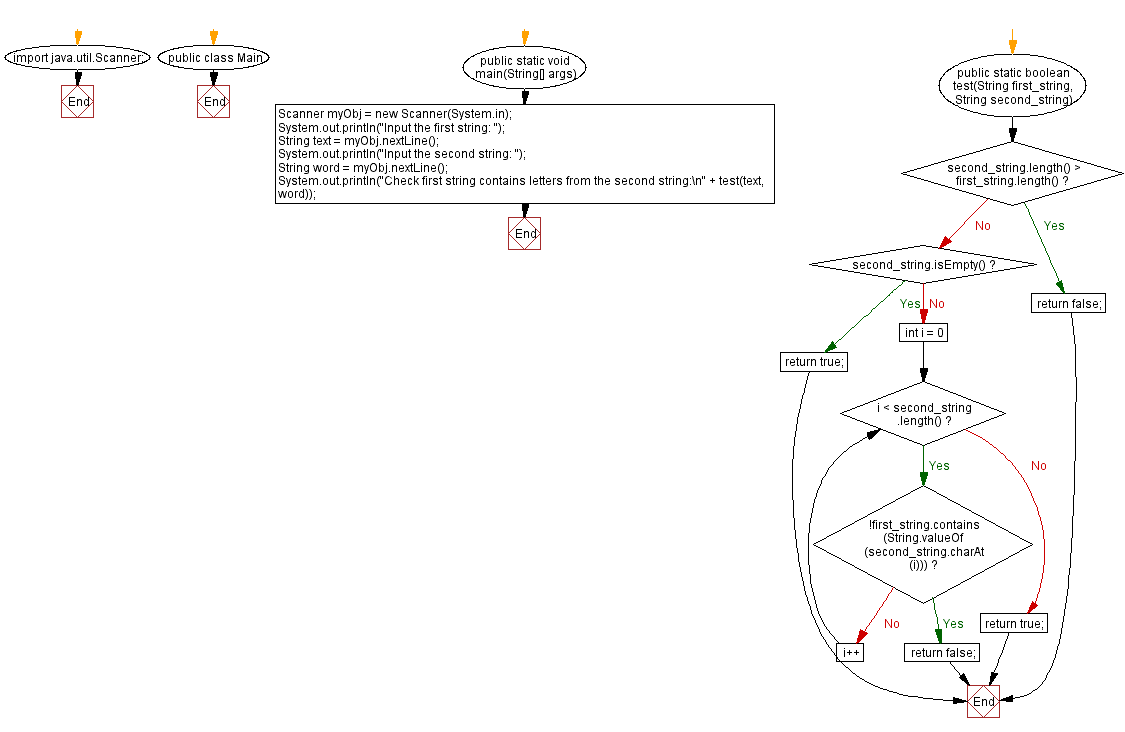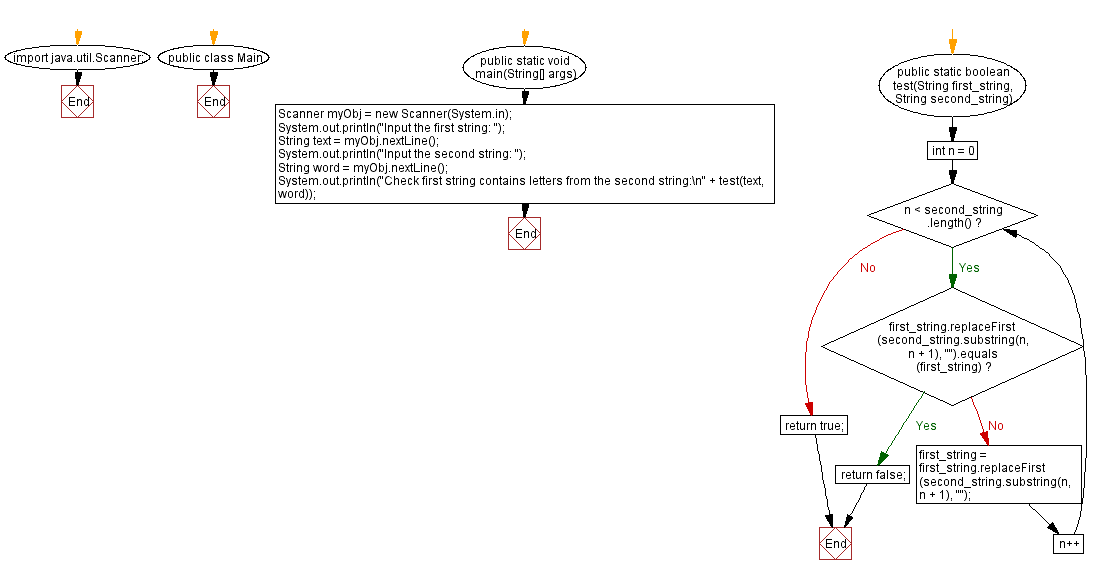﻿ Java - Check first string contains letters from the second

# Java: Check first string contains letters from the second

## Java String: Exercise-112 with Solution

A string is created by using another string's letters. Letters are case sensitive. Write a Java program that checks the letters of the second string are present in the first string. Return true otherwise false.

Visual Presentation:Sample Data:

("Python", "python") -> false
("Java", "Ja") -> true
("Check first string", "sifC") ->true

Sample Solution-1:

Java Code:

``````import java.util.Scanner;

public class Main {
public static void main(String[] args) {
Scanner myObj = new Scanner(System.in); // Create a Scanner object for user input

// Prompt the user to input the first and second strings
System.out.println("Input the first string: ");
String text = myObj.nextLine(); // Read the first string
System.out.println("Input the second string: ");
String word = myObj.nextLine(); // Read the second string

// Check if the first string contains all letters from the second string
System.out.println("Check first string contains letters from the second string:\n" + test(text, word));
}

// Method to check if the first string contains all letters from the second string
public static boolean test(String first_string, String second_string) {
if (second_string.length() > first_string.length()) return false; // If the second string is longer, return false
if (second_string.isEmpty()) return true; // If the second string is empty, return true

// Iterate through each character in the second string
for (int i = 0; i < second_string.length(); i++) {
// If the first string does not contain the current character from the second string, return false
if (!first_string.contains(String.valueOf(second_string.charAt(i)))) {
return false;
}
}
return true; // Return true if all characters from the second string are found in the first string
}
}
``````

Sample Output:

```Input the first string:
Java
Input the second string:
Ja
Check first string contains letters from the second string:
true
```

Flowchart:Sample Solution-2:

Java Code:

``````import java.util.Scanner;

public class Main {
public static void main(String[] args) {
Scanner myObj = new Scanner(System.in); // Create a Scanner object for user input

// Prompt the user to input the first and second strings
System.out.println("Input the first string: ");
String text = myObj.nextLine(); // Read the first string
System.out.println("Input the second string: ");
String word = myObj.nextLine(); // Read the second string

// Check if the first string contains all letters from the second string
System.out.println("Check first string contains letters from the second string:\n" + test(text, word));
}

// Method to check if the first string contains all letters from the second string
public static boolean test(String first_string, String second_string) {
for (int n = 0; n < second_string.length(); n++) // Loop through each character of the second string
if (first_string.replaceFirst(second_string.substring(n, n + 1), "").equals(first_string)) // If the character from the second string is not found in the first string
return false; // Return false
else
first_string = first_string.replaceFirst(second_string.substring(n, n + 1), ""); // Remove the character from the first string
return true; // Return true if all characters from the second string are found in the first string
}
}
``````

Sample Output:

```Input the first string:
Java
Input the second string:
Ja
Check first string contains letters from the second string:
true
```

Flowchart:Java Code Editor:

Improve this sample solution and post your code through Disqus

Previous Java Exercise: Remove a word from a given text.
Next Java Exercise: Java Date, Time and Calendar Exercises

What is the difficulty level of this exercise?

Test your Programming skills with w3resource's quiz.

﻿